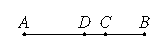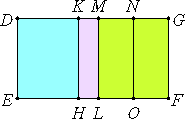# Lemma.

If a straight line is cut into unequal parts, then the sum of the squares on the unequal parts is greater than twice the rectangle contained by the unequal parts.

Let AB be a straight line, and let it be cut into unequal parts at C, and let AC be the greater.

I say that the sum of the squares on AC and CB is greater than twice the rectangle AC by CB.

Bisect AB at D.II.5

Since a straight line is cut into equal parts at D and into unequal parts at C, therefore the rectangle AC by CB together with the square on CD equals the square on AD, so that the rectangle AC by CB is less than the square on AD. Therefore twice the rectangle AC by CB is less than double the square on AD.

II.9

But the sum of the squares on AC and CB is double the sum of the squares on AD and DC, therefore the sum of the squares on AC and CB is greater than twice the rectangle AC by CB.

Q.E.D.

# Proposition 60

The square on the binomial straight line applied to a rational straight line produces as breadth the first binomial.

Let AB be a binomial straight line divided into its terms at C, so that AC is the greater term, let a rational straight line DE be set out, and let DEFG equal the square on AB be applied to DE producing DG as its breadth.

I say that DG is a first binomial straight line.Apply to DE the rectangle DH equal to the square on AC, and KL equal to the square on BC. Then the remainder, twice the rectangle AC by CB, equals MF.

Bisect MG at N, and draw NO parallel to ML or GF. Then each of the rectangles MO and NF equals once the rectangle AC by CB.

X.36

Now, since AB is a binomial divided into its terms at C, therefore AC and CB are rational straight lines commensurable in square only.

X.15

Therefore the squares on AC and CB are rational and commensurable with one an other, so that the sum of the squares on AC and CB is also rational. And it equals DL, therefore DL is rational.

X.20

And it is applied to the rational straight line DE, therefore DM is rational and commensurable in length with DE.

X.21

Again, since AC and CB are rational straight lines commensurable in square only, therefore twice the rectangle AC by CB, that is MF, is medial.

X.22

And it is applied to the rational straight line ML, therefore MG is also rational and incommensurable in length with ML, that is, DE.

X.13

But MD is also rational and is commensurable in length with DE, therefore DM is incommensurable in length with MG.

X.36

And they are rational, therefore DM and MG are rational straight lines commensurable in square only. Therefore DG is binomial.

It is next to be proved that it is also a first binomial straight line.

X.54,Lemma

Since the rectangle AC by CB is a mean proportional between the squares on AC and CB, therefore MO is also a mean proportional between DH and KL.

Therefore DH is to MO as MO is to KL, that is DK is to MN as MN is to MK. Therefore the rectangle DK by KM equals the square on MN.

Since the square on AC is commensurable with the square on CB, therefore DH is also commensurable with KL, so that DK is also commensurable with KM.

Since the sum of the squares on AC and CB is greater than twice the rectangle AC by CB, therefore DL is also greater than MF, so that DM is also greater than MG.

And the rectangle DK by KM equals the square on MN, that is, to the fourth part of the square on MG, and DK is commensurable with KM.

X.17

But, if there are two unequal straight lines, and to the greater there is applied a parallelogram equal to the fourth part of the square on the less and deficient by a square figure, and if it divides it into commensurable parts, then the square on the greater is greater than the square on the less by the square on a straight line commensurable with the greater. Therefore the square on DM is greater than the square on MG by the square on a straight line commensurable with DM.

And DM and MG are rational, and DM, which is the greater term, is commensurable in length with the rational straight line DE set out.

X.Def.II.1

Therefore DG is a first binomial straight line.

Therefore, the square on the binomial straight line applied to a rational straight line produces as breadth the first binomial.

Q.E.D.

## Guide

This proposition is used in X.72 and X.111.